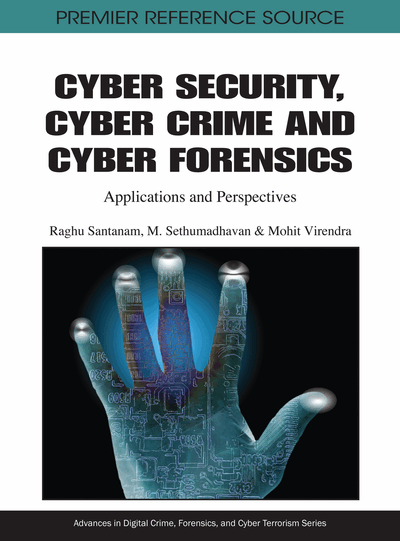# Error Linear Complexity Measures of Binary Multisequences

M. Sindhu, S. Kumar Sajan, M. Sethumadhavan
DOI: 10.4018/978-1-60960-123-2.ch017
OnDemand:
(Individual Chapters)
Available
\$37.50
No Current Special Offers

## Abstract

The joint linear complexity and k - error joint linear complexity of an m - fold 2n periodic multisequence can be efficiently computed using Modified Games Chan algorithm and Extended Stamp Martin Algorithm respectively. In this chapter the authors derive an algorithm which, given a constant c and an m – fold 2n periodic binary multisequence S, computes the minimum number k of errors and the associated error multisequence needed over a period of S for bringing the joint linear complexity of S below c . They derived another algorithm for finding the joint linear complexity of 3. 2v periodic binary multisequence.
Chapter Preview
Top

## Introduction

In complexity measures for sequences over finite fields, such as the linear complexity and the k – error linear complexity is of great relevance to cryptology, in particular, to the area of stream ciphers. There are basically two types of requirements for suitability of a keystream generated by a stream cipher. One of the requirements is the keystream sequence must pass various statistical tests for randomness. This is to make it difficult to capture any information about the plaintext by an attacker from any possible statistical deficiencies in the keystream. The second is that it should be very hard to predict the entire keystream from the knowledge of a part of it. For this purpose, one is interested to know how hard a sequence might be to predict. This requirement leads to the study of several complexity measures for sequences. The most significant complexity measure is the linear complexity: length of the shortest linear recurrence relation satisfied by the sequence and related concept of k-error linear complexity (see Kaida, T. (1999)).

The major problem in stream cipher cryptography is generating a pseudorandom sequence of elements from a short random key. We mainly use LFSRs for the construction of stream ciphers. On the other hand, LFSRs can also be used to mount attacks on stream cipher systems. This attack is based on the Berlekamp-Massey algorithm: given a sequence S with terms in a finite field Fq of length n, the Berlekamp-Massey algorithm computes the feedback polynomial of the shortest LFSR that can generate the sequence S in O(n2) time. In fact, if the shortest LFSR is of length ℓ, then the algorithm needs just 2ℓ consecutive terms of the output sequence to determine its feedback polynomial. So this algorithm forms a universal attack on keystream generators since it carries the potential of substituting any keystream generator by its shortest linear equivalent. This leads to the concept of linear complexity of sequences. For a stream cipher system to be secure against the Berlekamp-Massey attack, it must not be possible to approximate the keystream sequence closely with a sequence of significantly smaller linear complexity. This means that changing a few terms in the keystream sequence must not cause a significant decrease of the linear complexity. This requirement leads to the concept of k-error linear complexity.

To take advantage of the general purpose processing units employed in the present day computers over public/private networks, we need the keystream to be a sequence of a few bytes, instead of bits. Such keystream generators are better suited for software implementation, and provide high throughput. Such ciphers are called word based (or vectorized) ciphers since they produce more than one bit per clock cycle. The theory of such stream ciphers requires the study of the complexity measures for multisequences, i.e., for parallel streams of finitely many sequences. In this direction, the joint linear complexity and the joint linear complexity profile of multisequences have been investigated. The theory of k-error joint linear complexity of multisequences has been investigated recently.

An m fold N periodic multisequence S can be interpreted as an m × N matrix over Fq. For defining the k-error joint linear complexity of multisequences, we need the following definitions of term distance and column distance.

1.1 Definition: Let S=(S(1), S(2),…,S(m)) and T=(T(1), T(2),…,T(m)) be two m fold N periodic multisequences over Fq. We define the term distance δT(S,T) between S and T as the number of entries in S that are different from the corresponding entries in T, and the column distance δC(S,T) as the number of columns in S that are different from the corresponding columns in T. We define the individual distance vector by δV(S, T) = (δ1, δ2, …, δm), where δj is the Hamming distance between the jth rows of S and T for 1 ≤ jm.

## Complete Chapter List

Search this Book:
Reset